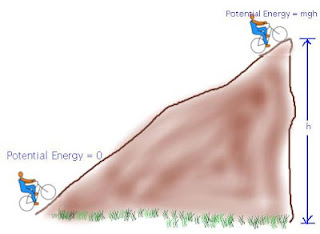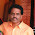Sunday, 7 October 2012

CBSE Class 9 - CH 11 - Work and Energy (Worksheet)

Work and Energy
Worksheet- Fill in the blanks

1. A force acting on a body shows a displacement in the direction normal to the direction of the force. It is said _______ done on the body.

2. A force acting on a body shows a displacement in the direction of the force. It is said _______ is done on the body.

3. Work done is defined as the product of  _____ and ______ in the direction of force.

4. SI Unit of work is  _______.

5. 1 Joule =   ____ × ____.

6.  Work done on an object is _____ if displacement is zero.

7.  Work is a ______ (scalar/vector)  quantity.

8.  Work done is positive when the angle between force and displacement is ____ (acute/obtuse)

9.  Work done is negative when the angle between force and displacement is ____ (acute/obtuse)

10. An object moving in a circular orbit. The work done by the centrepetal force acting on the object is ______ (zero/non-zero).

11. Work done against a force is stored as _____.

12. Ability to do work is called _______.

13. Ability of a body to do work due to its position or configuration is known as _________ of the body.

14. A form of energy an object has because of its motion is called ________.

15. A stretched rubber band acquires _________(potential/kinetic) energy.

16. Work done against gravity in lifting a mass is stored as ______. Numerically, it is
equal to m ×_ ×__.

17. Work done by gravity on a falling body is available as ______ (kinetic/potential) energy on reaching the ground.

18. Kinetic Energy = ½ × _ × _

19. If the mass is doubled while its speed remains the same, the kinetic energy will be _____ (be same/double/four times) of the initial kinetic energy.

20. The sum of P.E. and K.E. is called __________.

1. work is not
2. work
3. force and displacement
4. Joules
5. 1 Newton × 1metre
6. zero
7. scalar
8. acute
9. obtuse
10. zero.
11. energy
12. energy
13. potential energy
14. kinetic energy
15. potential energy
16. potential energy, m×g×h
17. kinetic
18. ½ m v2
19. double
20. mechanical energy

.1.2.Try a little tougher.

3.Try a little tougher.

4.Try a little tougher

5.Try a little tougher